Two angle are supplementary if they add up to 180 degrees

Thus,
Solution:
6. Find the angle t such that t and the angle 150 50' 23'' are supplementary.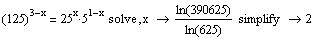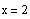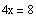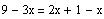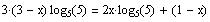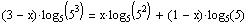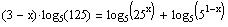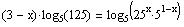Solution: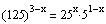5. Find the solution of the equation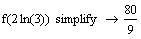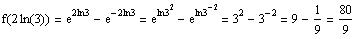Solution:
4. If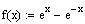, find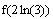Therefore, the range is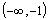Č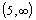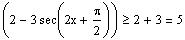or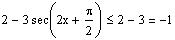or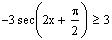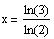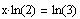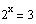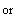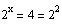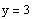or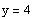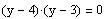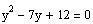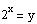Let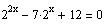Solution:
(Hint: simplify, then factor)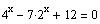8. Solve for x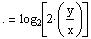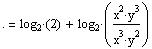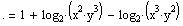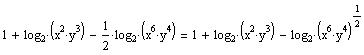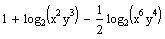Solution:
7. Let x and y be positive real numbers. Write down the following as a single logarithm:
t = 180 00' 00'' - 150 50' 23'' = 179 59' 60'' - 150 50' 23'' = 29 09' 37'''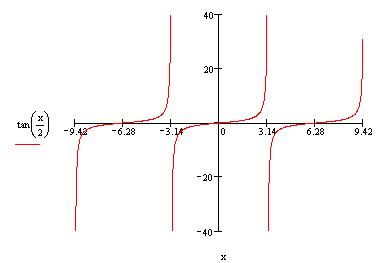Table 1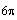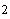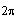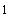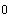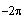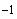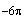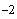---------------------------
x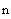So, in the interval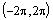there is on zero at x = 0.
The zero atandare not included since the interval is an open interval.

Figure 1 shows the graph of the function.
zero of the
function are at
Table 1 gives the zeros of the function for some choices of n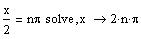The zero of tan(x) is at multiples of p. Thus,
Solution:
1. Find the number of zeros of the functionin the interval.
July 17, 2004
Major Quiz 7
Math002-033 A. Farhat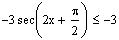or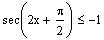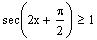Domain = R
Solution: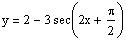3. Find the domain and range of the function
What is the period of the function?
<------------------------------------------------------------------ >
interval of interest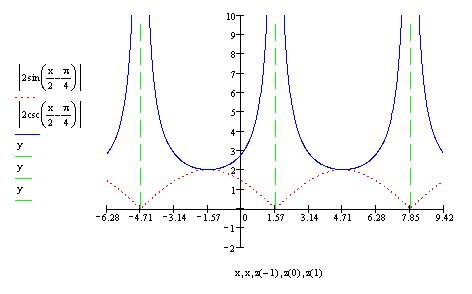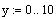Phase shift =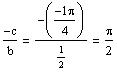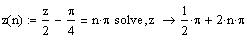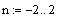The actual period is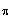because of the absolute value.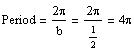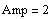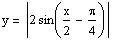The associated function is:
Solution: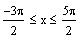for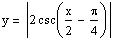2. Graph the function
Figure 1